Science, Maths & Technology

### Become an OU student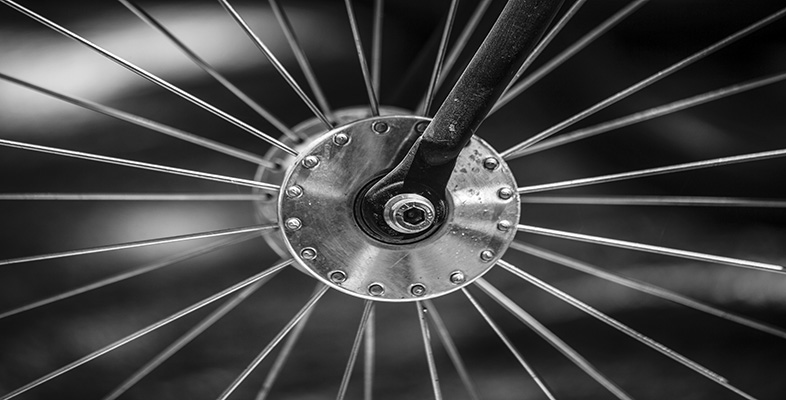Engineering: The nature of problems

Start this free course now. Just create an account and sign in. Enrol and complete the course for a free statement of participation or digital badge if available.

# 4.5.1 Mathematical models

Computers in the last few decades have, in many cases, made mathematical modelling a lot easier. Models that used to require hours of manual cranking through long equations can now be created on a screen using specialist software. Processes can be recreated – modelled – in the time it takes to press a few buttons.

For example, when designing a pipe network to carry a gas or fluid, such as in the village water supply problem, you might wish to know how the flow would be distributed within the network, i.e. at what rate the fluid would exit from each distribution point. In order to calculate this, you would need figures for: fluid density; the number of pipes in the system; the length and diameter of pipes joining each connector; the friction factor for each pipe (a constant determined by the roughness of the pipe wall); pressure losses at the pipe fittings; volumetric inflow at each connector; and so on. Imagine the calculations necessary to cover all of these, in what is actually a relatively uncomplicated arrangement of pipes.

Engineering has a whole host of branches, covering a huge variety of physical quantities. The following are examples of where computer-based techniques are used.

• Thermal and stress analyses model the distribution of quantities like temperature and stress across a shape or an area, and are useful for spotting potential weak points in a design.

• Computational fluid dynamic studies analyse turbulent flow in gases and liquids, such as in the simulation of weather.

• Universal Modelling Language simulates computer software.

• Circuit simulators model the performance of electronic circuits.

Of course, not all modelling is done on computers. Creating a mathematical model of something can be as simple as drawing a picture, putting in the mandatory dimensions and using the diagram or some simple calculations using algebra to determine the remaining quantities. For instance, look at the Box 8 Mathematical modelling example.

## Box 8 Mathematical modelling example

In another aspect of the village water supply problem outlined earlier, part of the design of the network may include some sort of storage tank. Let's assume that the tank is cylindrical and that it has been specified to be capable of holding 1500 litres (1.5 m3). In order to keep costs down, we want to use the minimum amount of material in constructing the tank and must therefore use mathematics to model the problem, finding the minimum surface area capable of holding the required volume.

The first step is to write down algebraic expressions for the key factors – volume and surface area in terms of the unknown quantities (radius r and length l of the cylinder) that are to be determined.

Volume: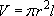Surface area (two ends plus the side):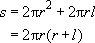Next, combine these expressions by using information from one equation to simplify the other.

Rearranging the equation for volume to express it in terms of length: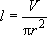We can substitute this expression for l into that for the surface area, bringing the problem down to one unknown, r, as the volume V is specified: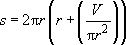Multiplying out and tidying up: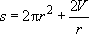There are two ways to find the minimum area for the required volume. The first is trial and error, for which a calculator or computer spreadsheet is invaluable. Try, for instance, a radius of 1 m and one of 2 m – which gives the smaller area? What value would you choose next? Try it. Often plotting a graph of the results helps you see where to direct your attention.

The second method is based on calculus, an analytical technique that should be familiar to you from your study of courses in mathematics. If it is not, you will have to stick to trial and error. For those who can follow it, the calculus approach is given below; otherwise move on to the text following Figure 13.

Differentiate the expression for the surface area with respect to r: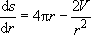For maxima and minima, ds/dr = 0 (remember that ds/dr is the gradient of the curve of s against r), so: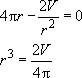Substituting the value V = 1.5 m3: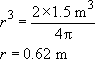We can use the earlier expression for the volume to find l: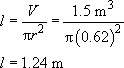Mathematical modelling tells us that the tank must be 1.24 m high with a radius of 0.62 m – that is, the length l is equal to the diameter (2r). Figure 13 shows how the shape of a cylinder looks as the ratio of r and l varies. It is obvious from our everyday experience that the cylinder which will hold the most water is the one that is neither extremely thin nor extremely short. Our numbers from the calculation look reasonable in this respect; if you looked at the cylinder side-on, it would look square.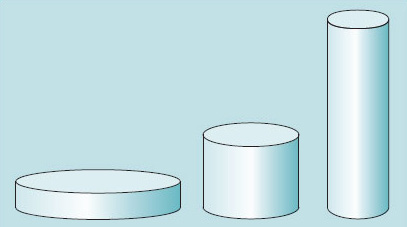Figure 13 Thin, 'square' and short cylinders with the same volume

## Exercise 3

In the mathematical modelling example we arrived at an 'optimum' cylinder which had a square cross-section (diameter equal to its length). But let's challenge this result by asking if any assumptions have led us away from the best solution.

A major assumption was made in setting up the problem, just before the mathematical modelling was presented. What was it? Do you think it was a reasonable one?

Can you think of reasons why the cylindrical shape may not be the best solution to this very specific problem?

Hint: think about the context and how this imposes constraints of its own.

The assumption was made that a cylindrical shape is going to be the best, and all we need to decide is the ratio of length (or height) to diameter. In fact, the shape that encloses the largest volume with the smallest surface is a sphere.

It seems reasonable to assume that, in this context, a spherical shape should not be used, as the problems of forming and joining or welding the sheet are considerable, and the necessary equipment is not likely to be available.

Here is my list of reasons why the cylinder may not be preferred – you may have thought of others:

• the shape of available sheet material

• the ability to cut circles and form the sheet into curves

• the space it has to fit in

• cultural preference for or aversion to a particular shape.

## SAQ 5

Making the cylindrical tank from a rectangular sheet of metal will create offcuts of material where the top and bottom circles have been cut out of the sheet. Show that if the most efficient cuboidal shape had been used instead (i.e. a cube), it would use less sheet material than the cylindrical shape. Assume that the width of the sheet material for each case is ideal.

To get a volume of 1.5 m3 in a cubic tank requires the side of the cube to be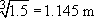. The cube has six faces, so this can be made from a sheet of material of dimensions 1.145 × (6 × 1.145) = 7.866.